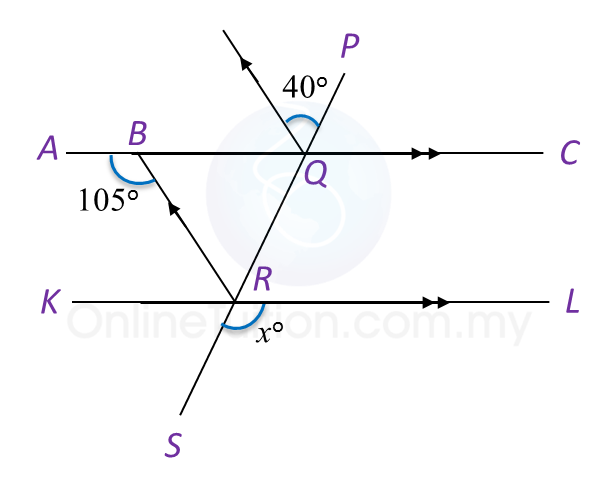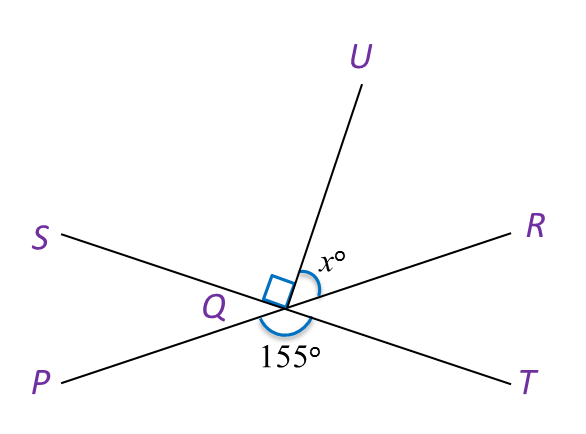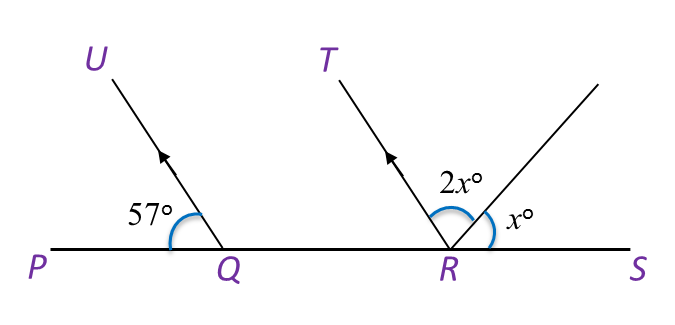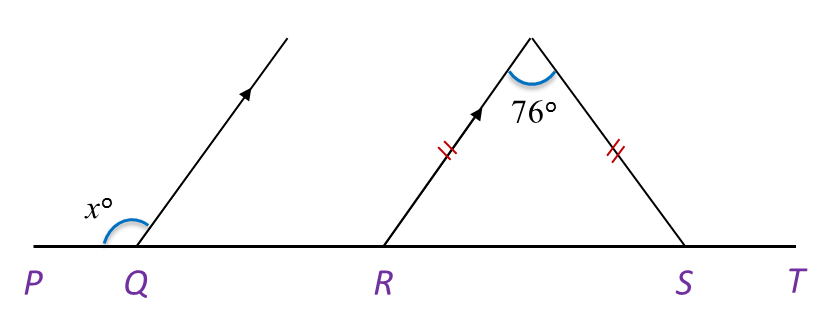# 8.2.1 Lines and Angles, PT3 Practice

 
 
8.2.1 Lines and Angles, PT3 Practice
 

 
Question 1:
 In Diagram below, PQRS, ABQC and KRL are straight lines.Find the value of x.
 
  Solution:$\begin{array}{l}\angle KRB+\angle ABR={180}^{o}←\overline{)\text{Sum of interior angles}}\\ \angle KRB+{105}^{o}={180}^{o}\\ \text{}\angle KRB={180}^{o}-{105}^{o}\\ \text{}\angle KRB={75}^{o}\\ \angle BRQ={40}^{o}←\overline{)\text{Corresponding angle}}\\ {x}^{o}=\angle SRL=\angle KRQ←\overline{)\begin{array}{l}\text{Vertically}\\ \text{opposite angle}\end{array}}\\ {x}^{o}={75}^{o}+{40}^{o}\\ \text{}={115}^{o}\\ \text{}x=115\end{array}$
 

 
 
 
 
Question 2:
 (a) In Diagram below, PQR and SQT are straight lines.Find the value of x.
 
 

  (b) In Diagram below, PQRS is a straight line. Find the value of x.Solution:
 
(a)
    $\begin{array}{l}\angle PQT=\angle RQS\\ {x}^{o}+{90}^{o}={155}^{o}\\ \text{}{x}^{o}={155}^{o}-{90}^{o}\\ \text{}={65}^{o}\\ \text{}x=65\end{array}$  
 
 

  (b)
    $\begin{array}{l}\angle QRT={57}^{o}\\ {x}^{o}+2{x}^{o}={180}^{o}-{57}^{o}\\ \text{3}{x}^{o}={123}^{o}\\ \text{}{x}^{o}=\frac{{123}^{o}}{3}\\ \text{}{x}^{o}={41}^{o}\\ \text{}x=41\end{array}$
 

 
 
Question 3:
In Diagram below, PQRST is a straight line. Find the value of x.Solution:

### 3 thoughts on “8.2.1 Lines and Angles, PT3 Practice”

1.NICE!!!!

2.tq..

3.this is sooooooooooooo useful but it would have been better if you explain